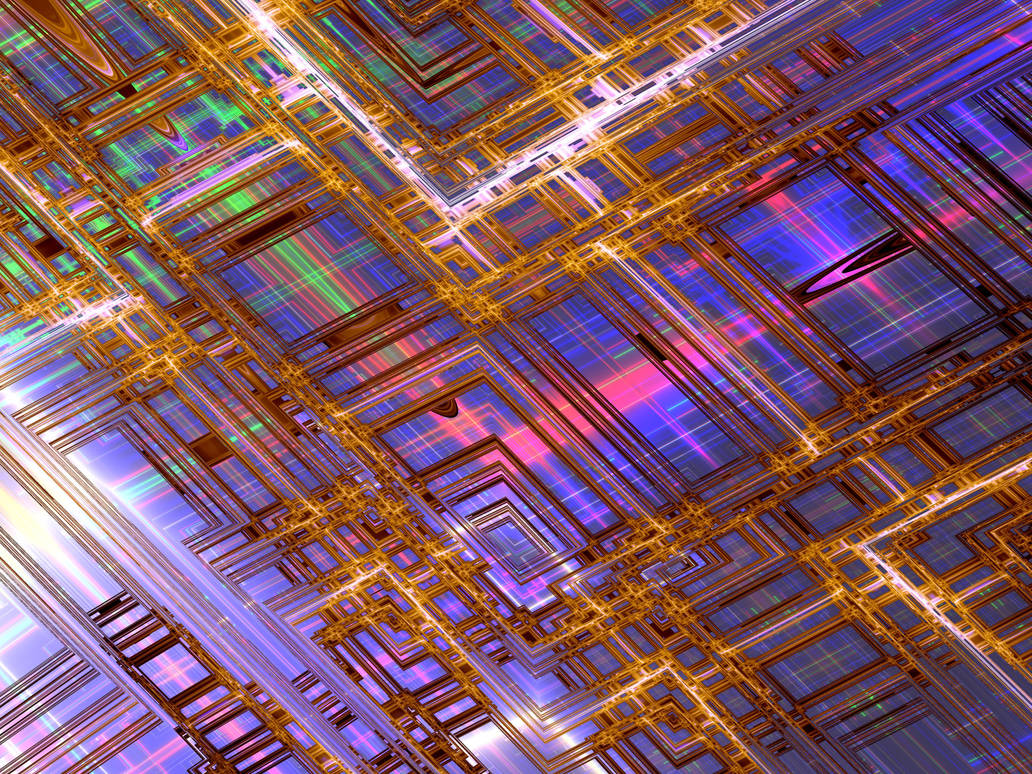• April 14, 2021, 06:12:00 AM

Login with username, password and session length

###Author Topic:  Zoomable square set  (Read 583 times)

0 Members and 1 Guest are viewing this topic.

####Alef##### Zoomable square set
« on: February 27, 2019, 05:07:06 PM »
This is nothing new. But I think it hadn't recieved deserved attention.

Pure split complex mandelbrot (i*i=1 ; i<>1) is just solid square. With few modifications it becomes zoomable complex geometric figure just like mandelbrot set but mostly based on right angles and parabolas.

Zuzubrot or Quadratic General formula. It need some better name than that.
x=abs( x*x+y*y +Cx )
y=abs( 2*x*y   +Cy )

With starting seed of (0.5,0) and bailout >= 50.

Ultra fractal parameter:
Code: [Select]
UF_Basic {fractal:  title="Fractal2" width=640 height=480 layers=1layer:  caption="Background" opacity=100mapping:  center=-0.5/0 magn=1formula:  maxiter=250 percheck=off filename="em.ufm" entry="Zuzubrot"  p_settype=Mandelbrot p_switchsettype=Julia p_bailout=128  p_inverted=no p_function=Abs p_talisfactor=0.4 p_julia=-1.6/0  p_start=0.5/0 f_func_post=identinside:  transfer=noneoutside:  transfer=lineargradient:  smooth=yes index=0 color=8716288 index=100 color=16121855 index=200  color=46591 index=300 color=156opacity:  smooth=no index=0 opacity=255}
This formula is pretty simple for Kalles Fractaler but it probably don't needs it's advanced capabilities.
No images just an artistic render.Linkback: https://fractalforums.org/index.php?topic=2653.0
catalisator of fractals

####claude##### Re: Zoomable square set
« Reply #1 on: February 27, 2019, 10:32:50 PM »
I rendered a slightly different formula (abs before instead of after each step) in 'et', coloured by atom domains and distance estimation.
Code: [Select]
z := ((x^2+y^2) + i |2xy|) + cAuto-skew was used to unstretch the 3rd image.  Seed was 0+0i.

If you're happy with the modification of the formula (KF needs a +c at the outside), decide on a name and I can add it to the next KF release, even though deep zooming might not be so interesting (I didn't find mini-sets yet).

###Similar Topics###### Trees Revisited square version

Started by Dinkydau on Fractal Image Gallery

0 Replies
319 ViewsNovember 03, 2017, 11:49:38 AM
by Dinkydau###### RSS feed item title contains HTML escape code instead of close square bracket

Started by claude on Discuss Fractalforums

2 Replies
545 ViewsSeptember 24, 2017, 05:22:35 PM
by Anon